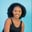Related Tags

python

# How to find the cumulative product of a 2D array in PythonMaria Elijah

## Overview

In Python, we can find the cumulative product of array elements using the cumprod() method from the NumPy library.

### The numpy.cumprod() method

The numpy.cumprod() method returns the cumulative product of elements in a given input array over a specified axis.

Note: A two-dimensional (2D) array can be created using a list of lists in Python.

### Syntax

numpy.cumprod(arr, axis=None, dtype=None, out=None)


### Parameters

• arr: This is the input array.
• axis: This is an optional parameter. It represents the axes along which the operation is to be performed.
• dtype: This is an optional parameter. It represents the return type of the array.
• out: This is an optional parameter. It represents the alternative output array in which the result is to be placed.

### Return value

The value returned is the cumulative product of the elements in the given input array over a specified axis.

Note: If the out parameter is specified, it returns an array reference to out.

### Code

The following code shows how to find the cumulative product of the two-dimensional (2D) array using the numpy.cumprod() method.

# import numpy
import numpy as np
# create a list
my_list1 = [24,8,3,4,34,8]
my_list2 = [5,7,2,10,15,7]
# convert to a numpy 2D array
np_list = np.array([my_list1, my_list2])
# compute the cummulative product of 2D array
# and store the result in np_list_cumprod
np_list_cumprod = np.cumprod(np_list, axis=0)

print(np_list_cumprod)
Cumulative product of a 2D array in Python

### Explanation

• Line 2: We import the numpy library.
• Lines 4–5: We create two lists, namely my_list1 and my_list2.
• Line 7: We convert the lists to a NumPy array which gives us a 2D array. We then store the value in a variable called np_list.
• Line 10: We use the np.cumprod() to compute the cumulative product of elements in the np_list and store it in np_list_cumprod.
• Line 11: We display the result.

RELATED TAGS

python

CONTRIBUTORMaria Elijah
RELATED COURSES

View all Courses

Keep Exploring

Learn in-demand tech skills in half the time